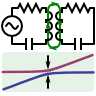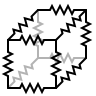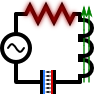Basic A.C. Circuits

We have seen how Maxwell's equations can be reduced to explain electro- and magnetostatic phenomena. In the employed descriptions, electric and magnetic field are independent of each other. In electrostatics we assumed a curl-free electric field, $$\nabla\times\mathbf{E}\left(\mathbf{r}\right)=0$$. This equation is now replaced by the so-called Maxwell-Faraday equation $\nabla\times\mathbf{E}\left(\mathbf{r},t\right) = -\partial_{t}\mathbf{B}\left(\mathbf{r},t\right)\$whereas the other equations, $$\nabla\cdot\mathbf{E}\left(\mathbf{r},t\right)=\rho\left(\mathbf{r},t\right)/\varepsilon_{0}$$, $$\nabla\times\mathbf{B}\left(\mathbf{r},t\right)=\mu_{0}\mathbf{j}\left(\mathbf{r},t\right)$$ and $$\nabla\cdot\mathbf{B}\left(\mathbf{r},t\right)=0$$ remain unchanged with respect to an explicit time dependency. Please note that we do not work with the full Maxwell eqquations yet. This version is often termed the slowly varying fields approximation of Maxwell's equations and neglects radiation contributions. This formulation is nevertheless extremely useful. Employing the modularization of electrical engineering boiling down electrodynamics to the interaction of the three basic passive elements resistor, capacitor, we will be able to understand a lot of basic phenomena. Thus, we will have a good starting point for more involved circuit theory aspects and a decent starting point for full electrodynamics.

Detuning in Coupled Oscillatory CircuitsWe all know them: twins. Either they love and support each other or they are fighting to find out who is the best. The reason is that they are almost equal. In this problem we will find out why they behave as they do understanding coupled oscillatory circuits.

The Resistance Cube, the C-Cube and the RLC-CubeTo simplify given circuits is an art of electrical engineers. Often it turns out that a complicated problem seen from a different point of view is extremely easy. The resistance cube ("R-cube") is one such example. The solution we will find can also be used to calculate the impedances of more complicated elements like the "C-cube" or the oscillatory "RLC-cube".

Dissipation in the Serial RLC CircuitWe will study one of the main problems of circuit theory and find basic concepts. Find out when the time-averaged dissipated power in the circuit is maximal using the phasor notation. Get to know a method to calculate the complex eigenfrequencies of the circuit.